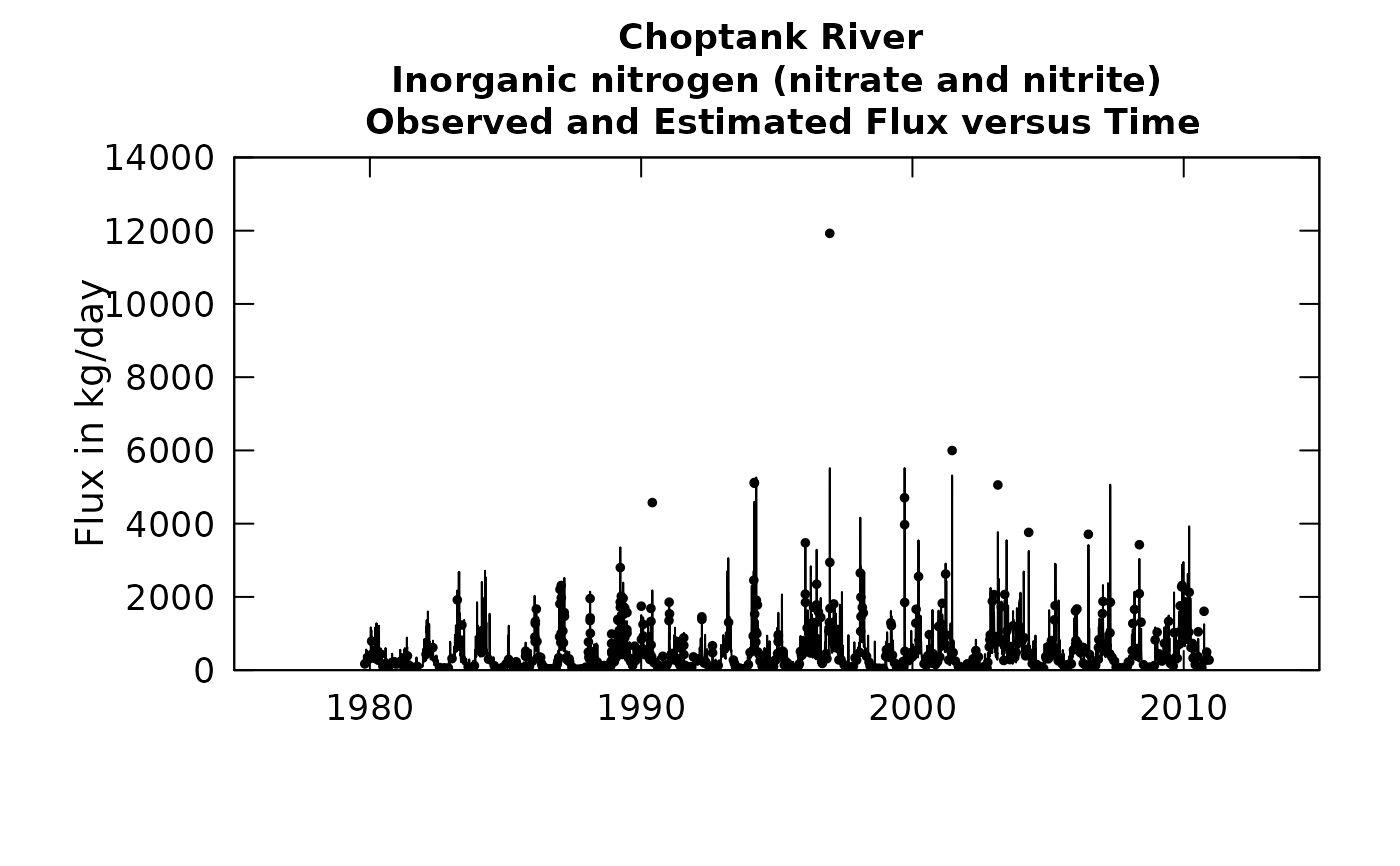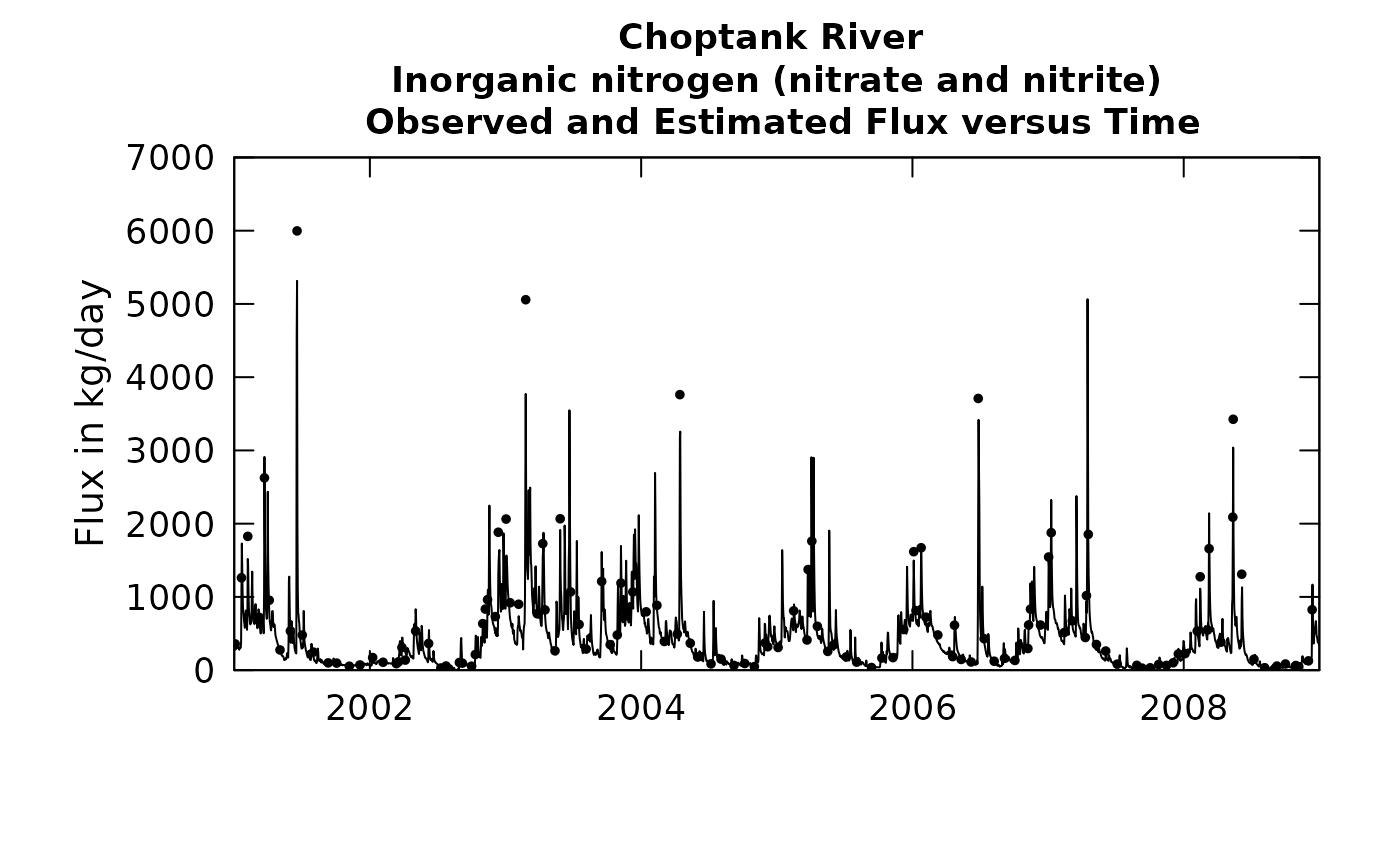This plot is useful for visual examination of the ability of the WRTDS, or other model, to fit the data, as seen in a time-series perspective.

Although there are a lot of optional arguments to this function, most are set to a logical default.

Data come from named list, which contains a Sample dataframe with the sample data, a Daily dataframe with the daily flow data, and an INFO dataframe with metadata.

## Usage

plotFluxTimeDaily(eList, yearStart = NA, yearEnd = NA, tinyPlot = FALSE,
fluxUnit = 3, fluxMax = NA, randomCensored = FALSE,
printTitle = TRUE, plotGenFlux = TRUE, usgsStyle = FALSE, cex = 0.8,
cex.axis = 1.1, cex.main = 1.1, customPar = FALSE, col = "black",
lwd = 1, prettyDate = TRUE, ...)

## Arguments

eList

named list with at least the Daily, Sample, and INFO dataframes

yearStart

numeric specifying the starting date (expressed as decimal years, for example 1989.0) for the plot

yearEnd

numeric specifying the ending date for the plot

tinyPlot

logical variable, if TRUE plot is designed to be short and wide, default is FALSE.

fluxUnit

number representing in pre-defined fluxUnit class array. printFluxUnitCheatSheet

fluxMax

number specifying the maximum value to be used on the vertical axis, default is NA (which allows it to be set automatically by the data)

randomCensored

logical, if TRUE plot a random value for censored data. Default is FALSE.

printTitle

logical variable if TRUE title is printed, if FALSE title is not printed (this is best for a multi-plot figure)

plotGenFlux

logical variable. If TRUE, annual flux points from WRTDSKalman output are plotted, if FALSE WRTDS flux is plotted.

usgsStyle

logical option to use USGS style guidelines. Setting this option to TRUE does NOT guarantee USGS compliance. It will only change automatically generated labels.

cex

numerical value giving the amount by which plotting symbols should be magnified

cex.axis

magnification to be used for axis annotation relative to the current setting of cex

cex.main

magnification to be used for main titles relative to the current setting of cex

customPar

logical defaults to FALSE. If TRUE, par() should be set by user before calling this function (for example, adjusting margins with par(mar=c(5,5,5,5))). If customPar FALSE, EGRET chooses the best margins depending on tinyPlot.

col

color of points on plot, see ?par 'Color Specification'

lwd

number line width

prettyDate

logical use 'pretty' limits for date axis if TRUE, or force the yearStart/yearEnd as limits if FALSE

...

arbitrary graphical parameters that will be passed to genericEGRETDotPlot function (see ?par for options)

selectDays, genericEGRETDotPlot

## Examples

eList <- Choptank_eList
# Water year:
plotFluxTimeDaily(eList)
#> plotGenFlux = TRUE requires running WRTDSKalman
#>               on eList. Switching to WRTDS concentration.plotFluxTimeDaily(eList, 2001,2009)
#> plotGenFlux = TRUE requires running WRTDSKalman
#>               on eList. Switching to WRTDS concentration.